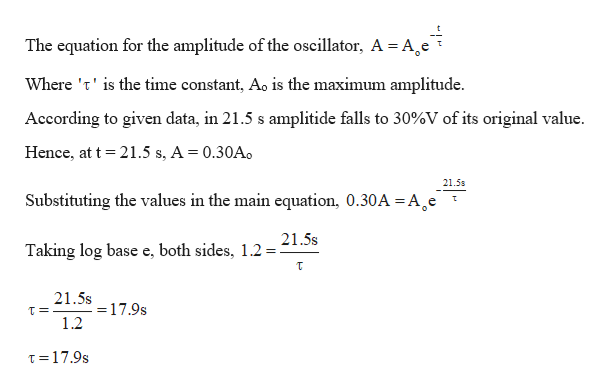# What is the time constant of an oscillator if its amplitude of oscillation is decreased to 30.0% of its original value in 21.5 s?- 35.7 s- 8.93 s- 17.9 s- 3.16 s

Question
14 views

What is the time constant of an oscillator if its amplitude of oscillation is decreased to 30.0% of its original value in 21.5 s?

- 35.7 s
- 8.93 s
- 17.9 s
- 3.16 s

check_circle

Step 1

Calculating the time-constan...help_outlineImage TranscriptioncloseThe equation for the amplitude of the oscillator, A = A eT Where 't' is the time constant, Ao is the maximum amplitude According to given data, in 21.5 s amplitide falls to 30%V of its original value Hence, at t 21.5 s, A = 0.30Ao 21.5s Substituting the values in the main equation, 0.30A =A^e 21.5s Taking log base e, both sides, 1.2 T 21.5s - 17.9s 1.2 T= T 17.9s fullscreen

### Want to see the full answer?

See Solution

#### Want to see this answer and more?

Solutions are written by subject experts who are available 24/7. Questions are typically answered within 1 hour.*

See Solution
*Response times may vary by subject and question.
Tagged in

### Other